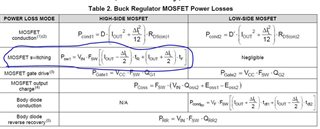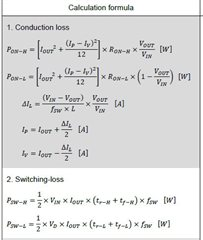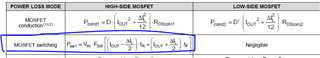If you have a related question, please click the "Ask a related question" button in the top right corner. The newly created question will be automatically linked to this question.

# LM5146-Q1: LM5146 MOSFET power dissipation worst case analysis

Part Number: LM5146-Q1
Other Parts Discussed in Thread: LM5146

Hello all

when I do a WCA with LM5146 ,I have some doubts about the datasheet :

the most important is about the MOSFET calculation formula :1. about the MOSFET calculation in LM5146 ,the MOSFET switching , Is the calculation formula correct ?
2. If the formula is not correct, I can use follow formula to analysis the MOSFET power dissipation?Thanks

• Hi huang,

The two sets of equations are effectively equivalent. The total switching transition time is the sum of the voltage / current rise and fall times (effectively a triangle of switching energy loss during high-side MOSFET turn on and turn off).

Regards,

Tim

• ok,I should use one of the last two formulas to calculate the switching loss .

mosfet switching loss  formula is not correct in LM5146 datasheet  ,is it right? thanks

• The equations are correct. TR and TF are the voltage rise and fall times -- the current transition times are assumed much smaller and thus neglected. You can crosscheck your power loss calculations with the LM5146 quickstart calculator.

Regards,

Tim

• Please look at that formula carefully in datasheet LM5146 ,Loss 1/2 .this is the main problem ...and We don't want to use this formula, but the premise is to make sure it's unreasonable

• Oh yes, there should be a factor of two in the expression. Thanks for pointing that out.

Regards,

Tim